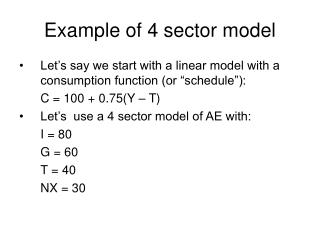Download PresentationExample of 4 sector modelExample of 4 sector model - PowerPoint PPT Presentation

Download PresentationExample of 4 sector model
An Image/Link below is provided (as is) to download presentation

Download Policy: Content on the Website is provided to you AS IS for your information and personal use and may not be sold / licensed / shared on other websites without getting consent from its author. While downloading, if for some reason you are not able to download a presentation, the publisher may have deleted the file from their server.

- - - - - - - - - - - - - - - - - - - - - - - - - - - E N D - - - - - - - - - - - - - - - - - - - - - - - - - - -
Presentation Transcript

1. Example of 4 sector model • Let’s say we start with a linear model with a consumption function (or “schedule”): C = 100 + 0.75(Y – T) • Let’s use a 4 sector model of AE with: I = 80 G = 60 T = 40 NX = 30

2. Firstly graph the consumption function. If we plug in 40 for T, we get (0.75*40 = 30): C = 100 + 0.75Y – 30 C = 70 + 0.75Y We know the vertical intercept (Y=0): C = 70 + 0.75 (0) = 70 Example of 4 sector model C C=70 + 0.75Y 70 Slope is 0.75 0 Y

3. Example of a 4 sector model • From the C function, we know our MPC is 0.75- a fact we will use later. • Now we need to derive our AE curve. We use the 4 sector AE equation: AE = C + I + G + NX • Plugging in the facts above, we get AE = 70 + 0.75Y + 80 + 60 + 30 I G NX C

4. Adding all those together, we get: AE = 240 + 0.75 Y Just like we did with the C function, we can graph this AE function: Example of a 4 sector model C, AE AE=240 + 0.75Y 240 C=70 + 0.75Y 70 0 Y

5. Example of a 4 sector model • Now we need to find the equilibrium of this model. This occurs where goods demand, AE, is equal to goods supply, Y. • Note that AE is a function of income, Y, and we are also requiring that in equilibrium goods supply, Y, is equal to AE. We are using Y in two different senses, as income and as output. But remember from the first lecture, we can calculate GDP either from income or from production and get the same value. • We are looking for an income level, Y*, that produces an AE level equal to Y*.

6. Using our equation for AE, we get: AE = 240 + 0.75Y* Y* = AE = 240 + 0.75Y* 0.25Y* = 240 Y* = 960 Example of a 4 sector model AE 45 deg line AE = 240 + 0.75Y 960 240 0 Y 960

7. Example of a 4 sector model • Since our MPC = 0.75, we know that the multiplier for autonomous expenditure is: Multiplier = 1/(1-MPC) = 1/(1-0.75) = 4 • So a \$1 change in I or G or NX would lead to a \$4 change in equilibrium GDP, Y*.